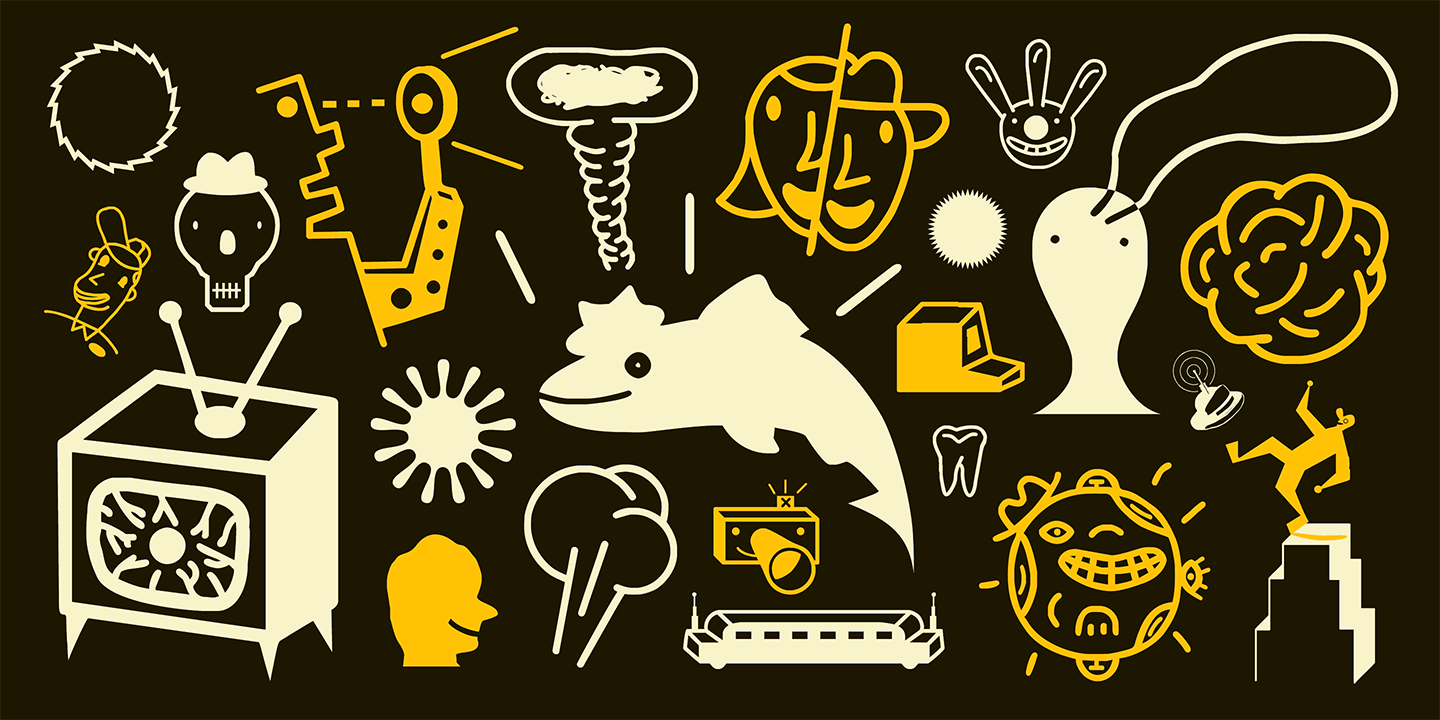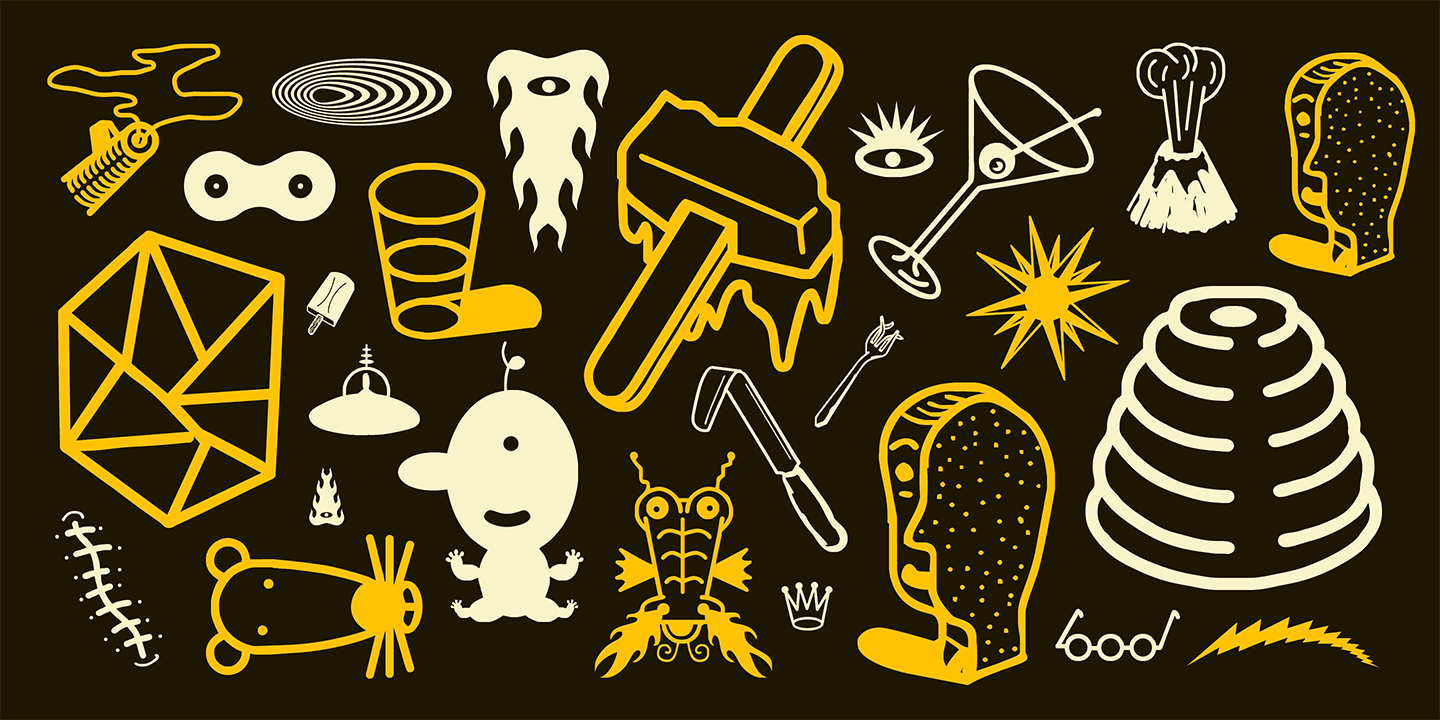# Thingbat

Designed by John Hersey in 1993.

A
A

## Thingbat (111 Illustrations) \$65.00

A a B b C c D d E e F f G g H h I i J j K k L l M m N n O o P p Q q R r S s T t U u V v W w X x Y y Z z

## Thingbat One

A a B b C c D d E e F f G g H h I i J j K k L l M m N n O o P p Q q R r S s T t U u V v W w X x Y y Z z